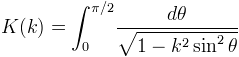﻿AdvancedMath.EllipticK Method

# AdvancedMathAddLanguageSpecificTextSet("LST6F5098A7_0?cpp=::|nu=.");EllipticK Method

Computes the complete elliptic integral of the first kind.

Namespace:  Meta.Numerics.Functions
Assembly:  Meta.Numerics (in Meta.Numerics.dll) Version: 4.0.5.0 (4.0.5.0)Syntax
```public static double EllipticK(
double k
)```

#### Parameters

k
Type: SystemDouble
The elliptic modulus, which must lie between zero and one.

#### Return Value

Type: Double
The value of the Legendre integral K(k).Exceptions
ExceptionCondition
ArgumentOutOfRangeExceptionk lies outside [0, 1].Remarks

K(k) is defined as the complete elliptic integral:It appears in the Legendre reduction of integrals of rational funtions.

Be aware that some authors use the the parameter m = k2 instead of the modulus k.See Also